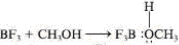Chapter 15.3, Problem 15.2EGeneral Chemistry - Standalone boo...

11th Edition
Steven D. Gammon + 7 others
ISBN: 9781305580343

Solutions

Chapter
SectionGeneral Chemistry - Standalone boo...

11th Edition
Steven D. Gammon + 7 others
ISBN: 9781305580343
Textbook Problem

Identify the Lewis acid and the Lewis base in each of the following reactions. Write the chemical equations using electron-dot formulas. a.b. O2− + CO2 →CO32−

Interpretation Introduction

Interpretation:

The Lewis acid and Lewis base in each of the given reactions and the chemical equations using electron-dot formulas has to be identified and written.

Concept introduction:

Lewis acid:

A species which can form a covalent bond by accepting electron pairs for the species is said to be Lewis acid.

Lewis base:

A species which can form a covalent bond by donating electron pairs for the species is said to be Lewis base.

Explanation

(a)

The Lewis acid and base in the given reaction is labeled as follows and electron-dot formula for the given reaction is represented as follows.

 + →

Still sussing out bartleby?

Check out a sample textbook solution.

See a sample solution

The Solution to Your Study Problems

Bartleby provides explanations to thousands of textbook problems written by our experts, many with advanced degrees!

Get Started

Alcohol is a natural substance and therefore does no real damage to body tissues. T F

Nutrition: Concepts and Controversies - Standalone book (MindTap Course List)

The smallest unit of life is the _____. a. atom b. molecule c. cell d. organism

Biology: The Unity and Diversity of Life (MindTap Course List)

How many autosomes are present in a body cell of a human being? In a gamete?

Human Heredity: Principles and Issues (MindTap Course List)

Explain the structure and function of the endomembrane system.

Biology: The Dynamic Science (MindTap Course List)